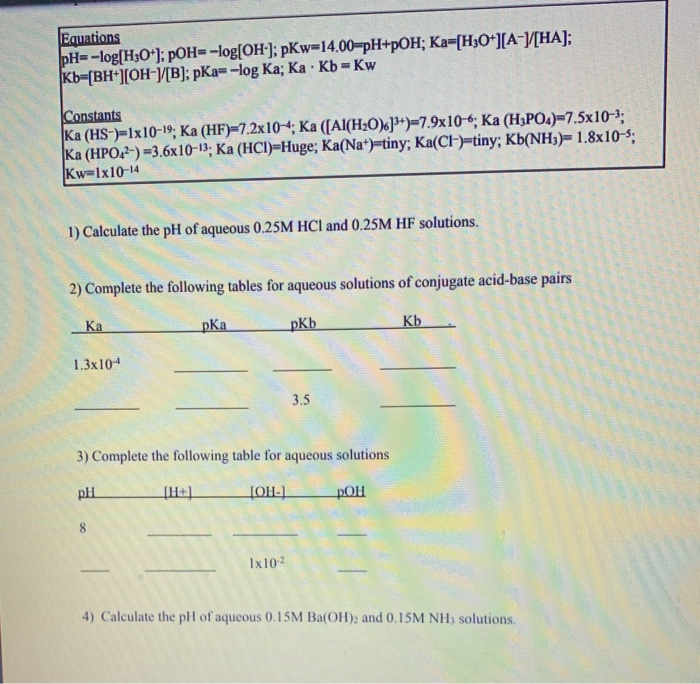Question

# The acid dissociation constant ka for an acid is 1x10-6 what is the pka? show the...

The acid dissociation constant ka for an acid is 1x10-6 what is the pka?

show the work

We need at least 10 more requests to produce the answer.

0 / 10 have requested this problem solution

The more requests, the faster the answer.

All students who have requested the answer will be notified once they are available.

#### Earn Coins

Coins can be redeemed for fabulous gifts.

Similar Homework Help Questions
• ### The acid dissociation constant, Ka, is 1x10-5 for a monoprotic mystery acid, HMyst. What is the...

The acid dissociation constant, Ka, is 1x10-5 for a monoprotic mystery acid, HMyst. What is the percent dissociation of a 0.10M HMyst? Show all work clearly so I can follow along please.

• ### The acid dissociation constant, Ka, is 1x10-5 for a monoprotic mystery acid, HMyst. What is the p...

The acid dissociation constant, Ka, is 1x10-5 for a monoprotic mystery acid, HMyst. What is the percent dissociation of a 0.10M HMyst? Show all work clearly so I can follow along please.

• ### If the equilibrium constant for the acid dissociation is KA and pKA (-log Ka) =7.0, what...

If the equilibrium constant for the acid dissociation is KA and pKA (-log Ka) =7.0, what is the ratio of [HPO42-] to [H2PO4−] in this buffer system? Please show your calculation.

• ### What is the pKa of an acid that has a Ka of 2.72*10^-4? please show all...

What is the pKa of an acid that has a Ka of 2.72*10^-4? please show all work :)

• ### A weak acid has a dissociation constant Ka = 2.5x10-2. Calculate the percentage dissociation for a...

A weak acid has a dissociation constant Ka = 2.5x10-2. Calculate the percentage dissociation for a 0.0750 m solution of this acid assuming a) ideal conditions and b) non-ideal conditions

• ### Calculate the acid dissociation constant Ka of a 0.5M solution of weak acid that is 0.3%...

Calculate the acid dissociation constant Ka of a 0.5M solution of weak acid that is 0.3% ionized.

• ### Equations pH=-log[H3O+]; pOH= -log[OH]; pKw=14.00=pH+POH; Ka-[H3O+][A-[HA]; Kb=[BH+][OH-)[B); pKa=-log Ka; Ka. Kb=Kw Constants Ka (HS-)=1x10-19; Ka (HF)...Equations pH=-log[H3O+]; pOH= -log[OH]; pKw=14.00=pH+POH; Ka-[H3O+][A-[HA]; Kb=[BH+][OH-)[B); pKa=-log Ka; Ka. Kb=Kw Constants Ka (HS-)=1x10-19; Ka (HF) 7.2x10-4; Ka ([Al(H20).]+)7.9x10-6; Ka (H3PO4)=7.5x10-2: Ka (HPO42-) =3.6x10-13; Ka (HCI)=Huge; Ka(Na+)=tiny; Ka(Cl-)tiny; Kb(NH3)=1.8x10-5; Kw=1x10-14 1) Calculate the pH of aqueous 0.25M HCl and 0.25M HF solutions. 2) Complete the following tables for aqueous solutions of conjugate acid-base pKa pKb Kb 1.3x10-4 35 3) Complete the following table for aqueous solutions DHL TH+) OH) pOH 1x102 4) Calculate the pH of aqueous 0.15M Ba(OH)2 and...

• ### HA(aq) is a weak acid with a dissociation constant, Ka, of 1.0 x 10-11. What is...

HA(aq) is a weak acid with a dissociation constant, Ka, of 1.0 x 10-11. What is the pH of a solution 0.055 M in A-(aq)? The temperature is 25oC.

• ### The acid dissociation constant Ka of boric acid H3BO3 is ×5.810−10. Calculate the pH of a...

The acid dissociation constant Ka of boric acid H3BO3 is ×5.810−10. Calculate the pH of a 3.1M solution of boric acid. Round your answer to 1 decimal place.

• ### The acid dissociation constant Ka of alloxanic acid HC4H3N2O5 is ×2.2410−7. Calculate the pH of a...

The acid dissociation constant Ka of alloxanic acid HC4H3N2O5 is ×2.2410−7. Calculate the pH of a 0.57M solution of alloxanic acid. Round your answer to 1 decimal place.